Next: A Dijkstra-based Selector Up: Results Previous: A Revised Connection Algorithm   Contents

## Applications to Isomerisation of LJ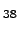and LJ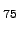As test cases for this algorithm we have considered various degenerate rearrangements of LJ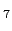, LJ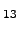, LJand LJ. (A degenerate rearrangement is one that links permutational isomers of the same structure [9,148].) In addition, we have considered rearrangements that link second lowest-energy structure with a global minimum for LJand LJclusters. The PES's of LJand LJhave been analysed in a number of previous studies [150,113,149,151], and are known to exhibit a double-funnel morphology: for both clusters the two lowest-energy minima are structurally distinct and well separated in configuration space. This makes them useful benchmarks for the above connection algorithm. Figure 2.9 depicts the energy profiles obtained using the revised connection algorithm for rearrangements between the two lowest minima of each cluster. In each case we have considered two distinct paths that link different permutational isomers of the minima in question, and these were chosen to be the permutations that give the shortest Euclidean distances. These paths will be identified using the distance between the two endpoints; for example, in the case of LJwe have paths LJ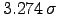and LJ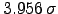, where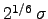is the pair equilibrium separation for the LJ potential.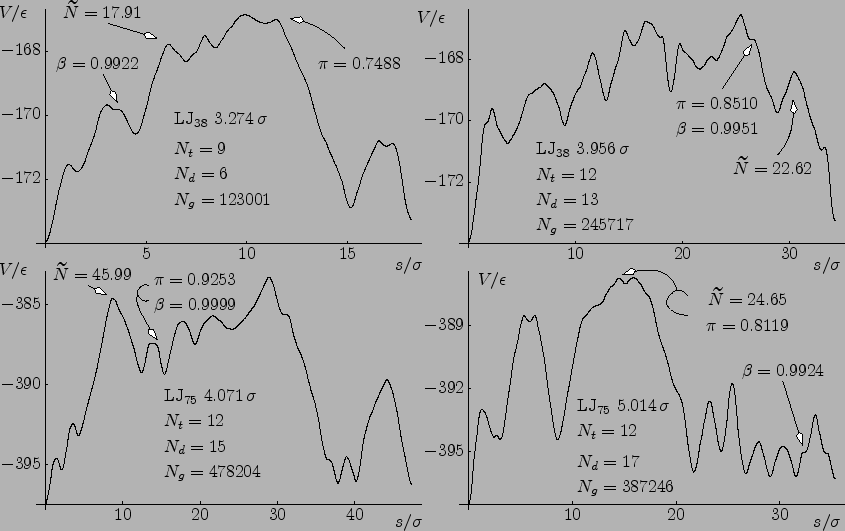For each calculation we used the following settings: the initial image density was set to 10, the iteration density to 30, and the maximum number of attempts to connect any pair of minima was limited to three. If a connection failed for a particular pair of minima then up to two more attempts were allowed before moving to the pair with the next smallest separation. For the second and third attempts the number of images was increased by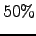each time. The maximum number of EF optimisation steps was set to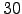with an RMS force convergence criterion of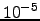. In Figure 2.9 every panel is labelled with the separation between the endpoints, the number of transition states in the final pathway, the number of DNEB runs required, and the total number of gradient calls.

Individual pathways involving a single transition state have been characterised using indices  such as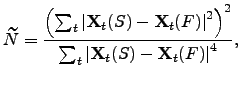(4.41)

which is a measure of the number of atoms that participate in the rearrangement. A modification of this Stillinger and Weber's participation index as well as a new cooperativity index will be presented in Chapter 3. Here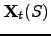and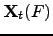are the position vectors of atom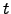in the starting and finishing geometries, respectively. The largest values are marked in Figure 2.9 next to the corresponding transition state. It is noteworthy that the pathways LJand LJ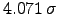both involve some highly cooperative steps, and the average value of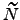is more than 12 for both of them.

We have found that it is usually easier to locate good transition state candidates for a multi-step path if the stationary points are separated by roughly equal distances, in terms of the integrated path length. Furthermore, it seems that more effort is needed to characterise a multi-step path when transition states involving very different path lengths are present. In such cases it is particularly beneficial to build up a complete path in stages. To further characterise this effect we introduce a path length asymmetry indexdefined as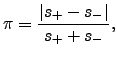(4.42)

where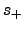and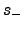are the two integrated path lengths corresponding to the two downhill steepest-descent paths from a given transition state. For example, in rearrangement LJ, five steps out of nine have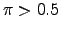.

Barrier asymmetry also plays a role in the accuracy of the tangent estimate, the image density required to resolve particular regions of the path, and in our selection process for transition state candidates, which is based on the condition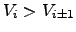. Unless there is no maximum in the profile, in which case we consider for transition state searching the highest energy image. To characterise this property we define a barrier asymmetry index,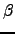, as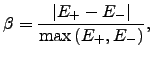(4.43)

where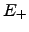and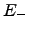are the barriers corresponding to the forward and reverse reactions, respectively. The test cases in Figure 2.9 include a variety of situations, with barrier asymmetry indexranging from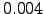to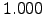. The maximum values ofandare shown next to the corresponding transition states in this figure.

We note that the total number of gradient evaluations required to produce the above paths could be reduced significantly by optimising the DNEB parameters or the connection strategy in each case. However, our objective was to find parameters that give reasonable results for a range of test cases, without further intervention.Next: A Dijkstra-based Selector Up: Results Previous: A Revised Connection Algorithm   Contents
Semen A Trygubenko 2006-04-10